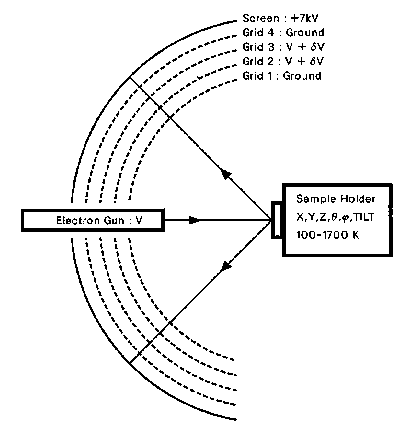Figure 2.1: Schematic LEED-AES retarding-field analyzer (RFA). A beam of mono-energetic electrons is emitted from the gun. Electrons diffract from the grounded sample surface. Backscattered electrons travel in a field-free region, between the sample and detector. Electrons are high-pass filtered by the 2nd and 3rd grids. Finally, the electrons excite a phosphor screen.

• Figure 1.8: The B_5 model for Si{111}rt3*rt3-30-X
• Figure 2.1: Schematic LEED-AES retarding-field analyzer (RFA).
• Figure 2.2: Schematic LEED pattern for Si{111} 1x1.
• Figure 2.3: Schematic LEED pattern for Si{111}rt3*rt3-30-X.
• Figure 2.4: A LEED pattern of Si{111}7*7, at 100 eV.
• Figure 2.5: Schematic LEED Data Acquisition System.
• Figure 2.6: Reliability-factor (R_VHT, r_ZJ, and R_P) contour plots for the surface of TB{11-20}.
• Figure 2.7: Schematic AES Data Acquisition System.
• Figure 2.8: Typical Auger electron spectrum for Au deposited upon a Si{111} surface.
• Figure 2.9: Schematic LEED-AES Surface Analysis UHV System.